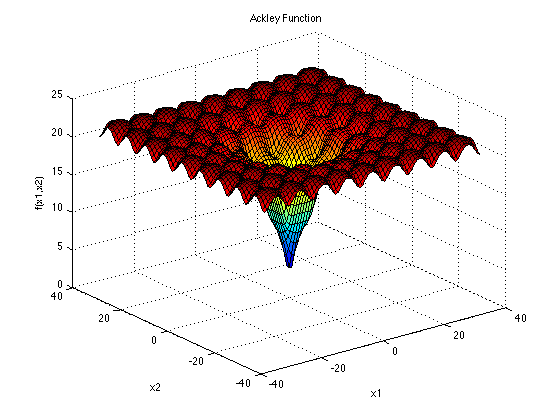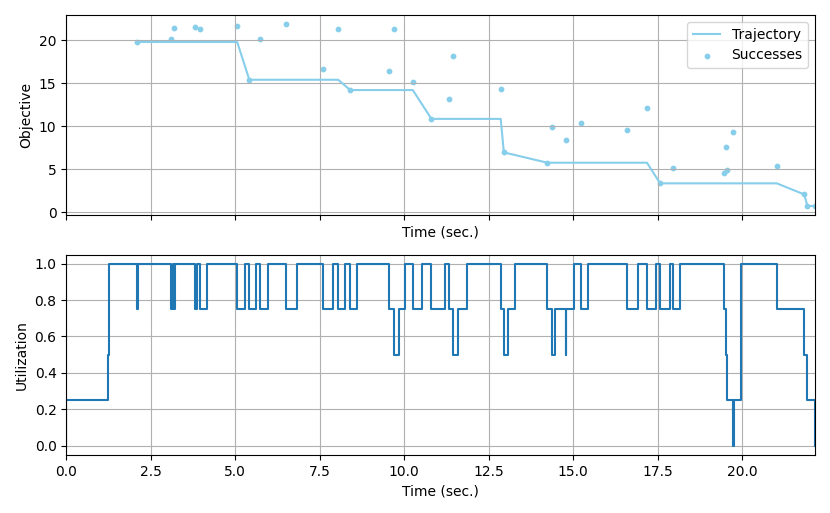# Profile the Worker Utilization#

Author(s): Romain Egele.

This example demonstrates the advantages of parallel evaluations over serial evaluations. We start by defining an artificial black-box `run`-function by using the Ackley function:We will use the `time.sleep` function to simulate a budget of 2 secondes of execution in average which helps illustrate the advantage of parallel evaluations. The `@profile` decorator is useful to collect starting/ending time of the `run`-function execution which help us know exactly when we are inside the black-box. This decorator is necessary when profiling the worker utilization. When using this decorator, the `run`-function will return a dictionnary with 2 new keys `"timestamp_start"` and `"timestamp_end"`. The `run`-function is defined in a separate module because of the “multiprocessing” backend that we are using in this example.

 ``` 1 2 3 4 5 6 7 8 9 10 11 12 13 14 15 16 17 18 19 20 21 22 23 24 25 26 27 28 29``` ```"""Set of Black-Box functions useful to build examples. """ import time import numpy as np from deephyper.evaluator import profile def ackley(x, a=20, b=0.2, c=2 * np.pi): d = len(x) s1 = np.sum(x**2) s2 = np.sum(np.cos(c * x)) term1 = -a * np.exp(-b * np.sqrt(s1 / d)) term2 = -np.exp(s2 / d) y = term1 + term2 + a + np.exp(1) return y @profile def run_ackley(config, sleep_loc=2, sleep_scale=0.5): # to simulate the computation of an expensive black-box if sleep_loc > 0: t_sleep = np.random.normal(loc=sleep_loc, scale=sleep_scale) t_sleep = max(t_sleep, 0) time.sleep(t_sleep) x = np.array([config[k] for k in config if "x" in k]) x = np.asarray_chkfinite(x) # ValueError if any NaN or Inf return -ackley(x) # maximisation is performed ```

After defining the black-box we can continue with the definition of our main script:

```import black_box_util as black_box
```

Then we define the variable(s) we want to optimize. For this problem we optimize Ackley in a 2-dimensional search space, the true minimul is located at `(0, 0)`.

```from deephyper.problem import HpProblem

nb_dim = 2
problem = HpProblem()
for i in range(nb_dim):
problem
```

Out:

```Configuration space object:
Hyperparameters:
x0, Type: UniformFloat, Range: [-32.768, 32.768], Default: 0.0
x1, Type: UniformFloat, Range: [-32.768, 32.768], Default: 0.0
```

Then we define a parallel search.

```if __name__ == "__main__":
from deephyper.evaluator import Evaluator
from deephyper.evaluator.callback import TqdmCallback
from deephyper.search.hps import CBO

timeout = 20
num_workers = 4
results = {}

evaluator = Evaluator.create(
black_box.run_ackley,
method="process",
method_kwargs={
"num_workers": num_workers,
"callbacks": [TqdmCallback()],
},
)
search = CBO(problem, evaluator, random_state=42)
results = search.search(timeout=timeout)
```

Out:

```0it [00:00, ?it/s]

1it [00:00, 11915.64it/s, objective=-21.5]

2it [00:00,  3.21it/s, objective=-21.5]

2it [00:00,  3.21it/s, objective=-21.2]

3it [00:01,  1.82it/s, objective=-21.2]

3it [00:01,  1.82it/s, objective=-20.6]

4it [00:01,  2.05it/s, objective=-20.6]

4it [00:01,  2.05it/s, objective=-20.6]

5it [00:03,  1.21it/s, objective=-20.6]

5it [00:03,  1.21it/s, objective=-19.9]

6it [00:03,  1.55it/s, objective=-19.9]

6it [00:03,  1.55it/s, objective=-19.9]

7it [00:04,  1.31it/s, objective=-19.9]

7it [00:04,  1.31it/s, objective=-19.9]

8it [00:05,  1.48it/s, objective=-19.9]

8it [00:05,  1.48it/s, objective=-19.9]

9it [00:06,  1.20it/s, objective=-19.9]

9it [00:06,  1.20it/s, objective=-19.9]

10it [00:06,  1.41it/s, objective=-19.9]

10it [00:06,  1.41it/s, objective=-19.9]

11it [00:07,  1.53it/s, objective=-19.9]

11it [00:07,  1.53it/s, objective=-19.9]

12it [00:08,  1.37it/s, objective=-19.9]

12it [00:08,  1.37it/s, objective=-19.9]

13it [00:10,  1.07s/it, objective=-19.9]

13it [00:10,  1.07s/it, objective=-11.3]

14it [00:10,  1.20it/s, objective=-11.3]

14it [00:10,  1.20it/s, objective=-11.3]

15it [00:10,  1.52it/s, objective=-11.3]

15it [00:10,  1.52it/s, objective=-11.3]

16it [00:11,  1.42it/s, objective=-11.3]

16it [00:11,  1.42it/s, objective=-11.3]

17it [00:13,  1.16s/it, objective=-11.3]

17it [00:13,  1.16s/it, objective=-11.3]

18it [00:13,  1.11it/s, objective=-11.3]

18it [00:13,  1.11it/s, objective=-10.7]

19it [00:13,  1.11it/s, objective=-10.7]

20it [00:14,  1.41it/s, objective=-10.7]

20it [00:14,  1.41it/s, objective=-10.5]

21it [00:15,  1.23it/s, objective=-10.5]

21it [00:15,  1.23it/s, objective=-9.85]

22it [00:16,  1.44it/s, objective=-9.85]

22it [00:16,  1.44it/s, objective=-9.85]

23it [00:17,  1.31it/s, objective=-9.85]

23it [00:17,  1.31it/s, objective=-9.85]

24it [00:17,  1.59it/s, objective=-9.85]

24it [00:17,  1.59it/s, objective=-9.85]
```

Finally, we plot the results from the collected DataFrame.

```if __name__ == "__main__":
import matplotlib.pyplot as plt
import numpy as np

def compile_profile(df):
"""Take the results dataframe as input and return the number of jobs running at a given timestamp."""
history = []

for _, row in df.iterrows():
history.append((row["timestamp_start"], 1))
history.append((row["timestamp_end"], -1))

history = sorted(history, key=lambda v: v)
nb_workers = 0
timestamp = 
n_jobs_running = 
for time, incr in history:
nb_workers += incr
timestamp.append(time)
n_jobs_running.append(nb_workers)

return timestamp, n_jobs_running

plt.figure()

plt.subplot(2, 1, 1)
plt.scatter(results.timestamp_end, results.objective)
plt.plot(results.timestamp_end, results.objective.cummax())
plt.xlabel("Time (sec.)")
plt.ylabel("Objective")
plt.grid()

plt.subplot(2, 1, 2)
x, y = compile_profile(results)
y = np.asarray(y) / num_workers * 100

plt.step(
x,
y,
where="pre",
)
plt.ylim(0, 100)
plt.xlabel("Time (sec.)")
plt.ylabel("Worker Utilization (%)")
plt.tight_layout()
plt.show()
```Total running time of the script: ( 0 minutes 20.101 seconds)

Gallery generated by Sphinx-Gallery